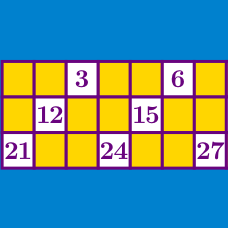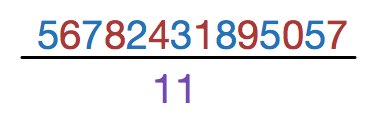Number Theory

# Divisibility: Level 1 Challenges

$\large \text{Is } 93123212 \text{ divisible by } 4?$Is 56782431895057 divisible by 11?

The digits 1, 2, 3, 4, and 5 are each used once to compose a five-digit number $\overline{ABCDE}$, such that the three-digit number $\overline{ABC}$ is divisible by 4, $\overline{BCD}$ is divisible by 5 and $\overline{CDE}$ is divisible by 3.

Submit the value of the five-digit number $\overline{ABCDE}$ as your answer.Can an even number, divided by another even number, times another even number ever equal an odd number?

If "yes," then find three numbers that work.
If "no," then why not?

Note that the three even numbers can be different numbers.

What digit, in place of the $\square$, will make the 8-digit integer, $2345678 \square$ a multiple of 6?

×